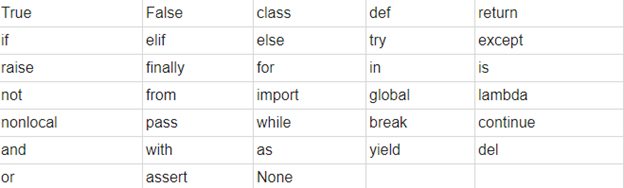## Python

Python is developed by Guido van Rossum in Dec 1989

Python is easy to use

Python is high level programming language

Python is open source language

## Tokens(Lexical unit)

The smallest unit of the program is called token.

#### The following are the tokens in Python.

Keywords,Identifiers (Names), Literals,Operators,Punctuators

#### Keywords:-

These are predefined reserve words that have special meaning to the language compiler or interpreter. These keywords we can not use as an identifier. Sometime it may be a command or parameter.#### Identifier (Names):-

A Python identifier is a name used to identify a variable, function, class, module, or another object.

Python does not allow punctuation characters such as @, \$, and % within identifiers. Python is a case sensitive programming language. Thus, System and system are two different identifiers in Python.

#### Here are naming conventions for Python identifiers −

• Starting an identifier with a single leading underscore indicates that the identifier is private.

• Starting an identifier with two leading underscores indicates a strongly private identifier.

• If the identifier also ends with two trailing underscores, the identifier is a language-defined special name.

Examples of Vaid Identifiers:

mystr, _test,Pie,PIE,Area_triangle

Examples of InValid Identifiers

2mystr, if ,test@,xyz\$

### Literals

Python literals are defined as data that is given in a variable or constant

Below are the supported literals in Python

#### 1. String Literals

String literals can be formed by enclosing a text in the quotes. We can use both single as well as double quotes to create a string

Example:

1.   "Raman" , '332'

Types of Strings:

There are two types of Strings supported in Python:

a) Single-line String- Strings that are terminated within a single-line are known as Single line Strings.

Example:

1.   text1='hello'

b) Multi-line String - A piece of text that is written in multiple lines is known as multiple lines string.

There are two ways to create multiline strings:

1) Adding black slash at the end of each line.

Example:

1.   text1='hello\

2.   India'

3.   print(text1)

'helloIndia'

2) Using triple quotation marks:-

Example:

1.   str2='''''welcome

2.   to

3.   India'''

4.   print str2

Output:

welcome

to

India

2. Numeric literals:

Numeric Literals are immutable. Numeric literals can belong to following four different numerical types.

 Int(signed integers) Long(long integers) float(floating point) Complex(complex) Numbers( can be both positive and negative) with no fractional part.eg: 100 Integers of unlimited size followed by lowercase or uppercase L eg: 87032845L Real numbers with both integer and fractional part eg: -26.2 In the form of a+bj where a forms the real part and b forms the imaginary part of the complex number. eg: 3.14j

Example - Numeric Literals

1.   x = 0b10100 #Binary Literals

2.   y = 100 #Decimal Literal

3.   z = 0o215 #Octal Literal

4.   u = 0x12d #Hexadecimal Literal

5.

6.   #Float Literal

7.   float_1 = 100.5

8.   float_2 = 1.5e2

9.

#Complex Literal

1             a = 5+3.14j

print(x, y, z, u)

print(float_1, float_2)

1    print(a, a.imag, a.real)

Output:

20 100 141 301

100.5 150.0

(5+3.14j) 3.14 5.0

3.Boolean literals:

A Boolean literal can have any of the two values: True or False.

Example - Boolean Literals

1.   x = (1 == True)

2.   y = (2 == False)

3.   z = (3 == True)

4.   a = True + 10

5.   b = False + 10

6.

7.   print("x is", x)

8.   print("y is", y)

9.   print("z is", z)

print("a:", a)

print("b:", b)

Output:

x is True

y is False

z is False

a: 11

b: 10

4. Special Literals

Python contains one special literal i.e., None.

None is used to specify to that field that is not created. It is also used for the end of lists in Python.

Example - Special Literals

1.   val1=10

2.   val2=None

3.   print(val1)

4.   print(val2)

Output:

10

None

5.Literal Collections.

Python provides the four types of literal collection such as List literals, Tuple literals, Dict literals, and Set literals.

List:

• List contains items of different data types. Lists are mutable i.e., modifiable.
• The values stored in List are separated by comma(,) and enclosed within square brackets([]). We can store different types of data in a List.

Example - List literals

1.   list=['John',678,20.4,'Peter']

2.   list1=[456,'Andrew']

3.   print(list)

4.   print(list + list1)

Output:

['John', 678, 20.4, 'Peter']

['John', 678, 20.4, 'Peter', 456, 'Andrew']

Dictionary:

• Python dictionary stores the data in the key-value pair.
• It is enclosed by curly-braces {} and each pair is separated by the commas(,).

Example

1.   dict = {'name''Pater''Age':18,'Roll_nu':101}

2.   print(dict)

Output:

{'name': 'Pater', 'Age': 18, 'Roll_nu': 101}

Tuple:

• Python tuple is a collection of different data-type. It is immutable which means it cannot be modified after creation.
• It is enclosed by the parentheses () and each element is separated by the comma(,).

Example

1.   tup = (10,20,"Dev",[2,3,4])

2.   print(tup)

Output:

(10, 20, 'Dev', [2, 3, 4])

Set:

• Python set is the collection of the unordered dataset.
• It is enclosed by the {} and each element is separated by the comma(,).

Example: - Set Literals

1.   set = {'apple','grapes','guava','papaya'}

2.   print(set)

Output:

{'guava', 'apple', 'papaya', 'grapes'}

#### Operators

Operators are used to triggering some computation when applied to variables or other objects in an expression.

Arithmetic Operators :- +,-,/,//,*,%

Bitwise Operators:- & , ^, |

Shift Operators:- >> <<

Identity Operators :- is , is not

relational operators:- >,<,>=,<=,!=

logical Operators:- and ,or, not

Membership Operators:- in, not in

Arithmetic Assignment:- /=,+=,*=,//=,

#### Punctators

These are the symbols that are used in the programming language to organize the sentence structure

eg

#,@,:,[]